## A constant force of 45 N directed at angle θ to the horizontal pulls a crate of weight 100 N from one end of a room to another a distance of

Question

A constant force of 45 N directed at angle θ to the horizontal pulls a crate of weight 100 N from one end of a room to another a distance of 4 m. Given that the vertical component of the pulling force is 12 N, calculate the work done by the force in moving the crate.

in progress 0
2 months 2021-07-31T15:02:14+00:00 1 Answers 4 views 0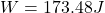Explanation:

information we know:

Total force: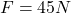Weight: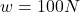distance: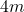vertical component of the force: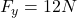————-

In this case we need the formulas to calculate the components of the force (because to calculate the work we need the horizontal component of the force).

horizontal component: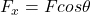vertical component: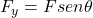but from the given information we know thatso, equation these twoand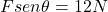and we know the force, thus: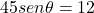now we clear for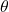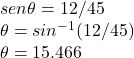the angle to the horizontal is 15.466°, with this information we can calculate the horizontal component of the force: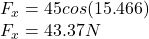whith this horizontal component we calculate the work to move the crate a distance of 4 m: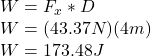the work done is W=173.48J